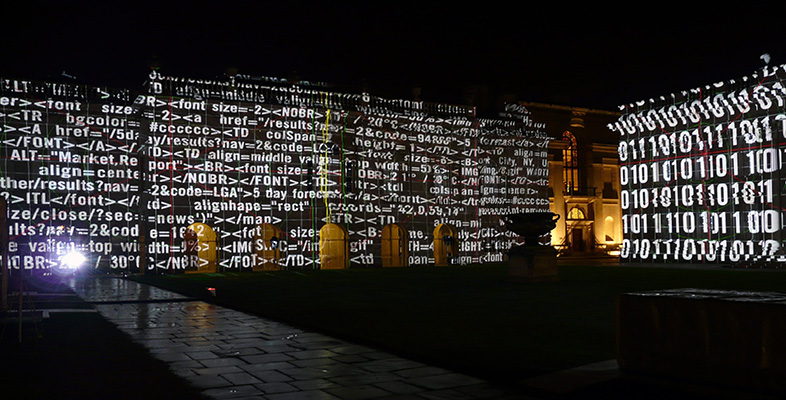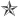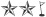Using numbers and handling data

Start this free course now. Just create an account and sign in. Enrol and complete the course for a free statement of participation or digital badge if available.

Free course

# 1 Decimals

## 1.1 Introducing the decimal system of numbers

Many different systems for writing numbers have been developed over the history of humankind.

The easiest way of counting small numbers is to use your fingers, and for this reason many numerical systems, such as the decimal system, are based around the number ten. But what happens when you run out of fingers to count on?

Numbering systems get round this problem by using a system of scale in which many small units are represented by a single larger unit, and many of these larger units are represented by a single even bigger unit, and so on.

For instance, the number five can be represented as five fingers, or by a single hand. In the same way, if you were writing numbers, you might want to use one symbol to represent single units and another to represent a larger collection of these units. How about a lineto represent an individual unit and a starto represent a collection of five individual units? Using this system, the number eleven could be represented as two stars and a line(i.e. five plus five plus one).

The decimal system uses a similar concept of scale, but it is arranged such that ten of any particular unit makes one unit of the next size up. This also works the other way round: each larger unit consists of ten units of the next size down.

For instance, ten pennies (ten 'units') are the same as one 10p piece (one 'ten'). Similarly, ten 10p pieces (in other words one hundred pennies) are the same as one pound.

These different groups differ by a factor of ten (each group is ten times larger or smaller than the one that precedes or follows it), which is also known as an order of magnitude (see Figure 1).

Groups of decimal numbers start from zero, so within a group of ten, any particular unit may be numbered between 0 and 9. Unlike many earlier systems of numbering, the decimal system doesn't rely on having to use different symbols to indicate larger and larger groupings of numbers. Instead, the order in which the symbols 0 to 9 are written provides the information that tells you the size of a number. In other words the decimal system is a positional system of numbering - the numbers are read and written from left to right, and the order in which they are written or spoken is used to tell you how many different factors of ten are present in the number. For example, the number 125 (one hundred and twenty five) contains three different orders of magnitude. On the left are the numbers of hundreds (one hundred), then the numbers of tens (two tens), and finally the numbers of units (five units).

The name of the unit of measurement is usually written to the right of the number, after a space, and this information tells you what individual objects are being counted (people, grams, metres, etc.). As such, a distance of one hundred and twenty five metres would be written as 125 m, where 'm' represents the unit of measurement.

S110_1